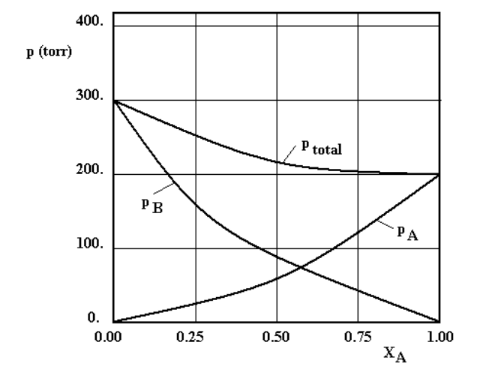# Problem: A and B are volatile miscible liquids. The partial pressures of A and B and the total pressure above a solution of A and B are given in the diagram below as a function of XA, the mole fraction of A, and at a temperature T = 40.0 °C. Based on the diagram answer the following questions.a) Do A and B form an ideal liquid solution (yes or no, and a brief justification for your answer)?b) What is pB°, the vapor pressure of pure B, at T = 40.0 °C?

###### FREE Expert Solution
85% (188 ratings)
###### Problem Details

A and B are volatile miscible liquids. The partial pressures of A and B and the total pressure above a solution of A and B are given in the diagram below as a function of XA, the mole fraction of A, and at a temperature T = 40.0 °C. Based on the diagram answer the following questions.

a) Do A and B form an ideal liquid solution (yes or no, and a brief justification for your answer)?

b) What is pB°, the vapor pressure of pure B, at T = 40.0 °C?